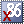# MatLab 5.x

MatLab is a high speed, interactive programming utility for manipulating, calculating, and plotting complicated mathematical equations. It is considered easy to use for those familiar with typical algebra equations.

It competed against Mathematica.

Early versions were copy protected.

Product type
Application Mathematics
Vendor
MathWorks
Release date
1999
User interface
GUI
Platform
WindowsMatlab R11 v5.3.0.10183 (1999) (ISO) R11 v5.3.0.10183 English407.37MB 3